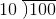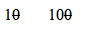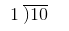# How to Divide Numbers Ending in Zeroes

Instructor: Sabrina Hairston

Sabrina has taught a variety of subjects and grades as a substitute teacher and will complete her MAT in 2016.

In this lesson, you will be taught how to divide numbers ending in zeros by learning how to simplify large problems by striking out the common zeros. Test your knowledge with the quiz at the end. Updated: 05/28/2020

## Zeros to the Rescue

Dividing large numbers can be intimidating, especially when they end in several zeros. Some of those large numbers may make you feel like you will never get to the end of the problem, but this does not have to be the case. Those zeros may actually be a reason to be thankful. Let's look at a couple of examples.

## How Zeros HelpOur first example tells us to divide 100 by 10. This example is not a hard one. The answer is 10. We know that 10 will go into 100 ten times. But, let's look at this problem another way. Another way you can divide numbers ending in zeros is to strike out the common zeros.

## Strike Out the Common Zeros

What does that mean? The common zeros are the zeros that both numbers have in common. For example, the number 100 and the number 10 each have one zero, so that zero is the one they have in common. We can only strike out one zero for each number because they only have one zero in common. So if we divide by striking out the common zeros, our numbers would now look like this:Notice, after we strike out the common zeros, we re-write the problem without the zeros that we made a strike through. Doing this changes our problem from 100 divided by 10, so that now our problem is 10 divided by 1.## Divide

Once the problem has been simplified by striking out the common zeros, it is time to divide. For our example problem, we are going to divide 10 by 1. One will go into one, one time. One will go into zero, zero times. Our answer is 10.

To unlock this lesson you must be a Study.com Member.

### Register to view this lesson

Are you a student or a teacher?